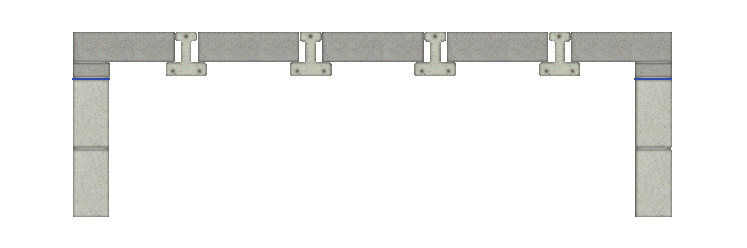The Floating Floor Estimating Calculator is used to calculate the labour and materials involved in constructing floating floors.The Floating Floor Estimating Calculator calculates the following items: